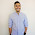## Saturday, January 5, 2013

### LIGHT

Isn't it amazing that light travels with a speed

which is equal to speed of light.

let me try to explain the difference between speed of light and the speed with which light travels.
While doing special relativity there appears a fundamental constant
say x whose value is equal to 3 * 10^8 and this constant has a dimension of LT^-1 ( m/ s )
Next we will use the famous Einstein's eqn.. m= M/ sqrt(1-v2/c2)
Now from this eqn since the rest mass of photons is 0, so it must travel with the speed equals to c, Now these photons are nothing but light, so we now know that light has to travel with the velocity . Of x which in turn is equal to 3 * 10^8 m/s, , Now u know that in some medium light can travel with speed much less than 3* 10^8 m/s

so let me sum up things - there is a fundamental constant whose value is equal to 3*10^8 m/s, Since light is nothing but mass less photon so light travels with speed equal to 3*10^8 m/s.

1.1.